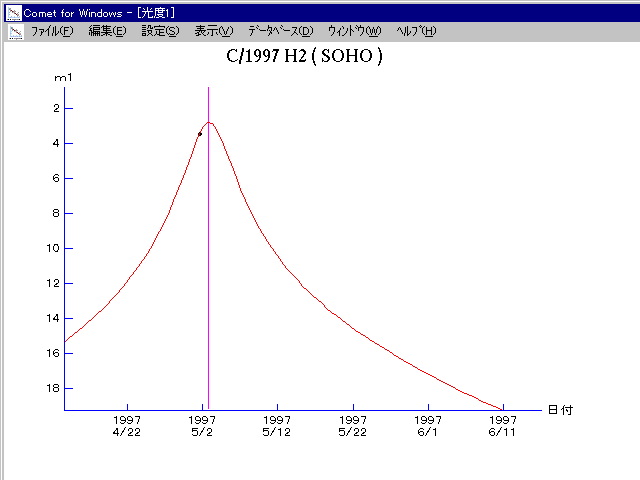# \$B%=!<%[!

C/1997 H2 ( SOHO )###\$B%W%m%U%#!<%k(B

 \$BH/8+F|(B 1997\$BG/(B4\$B7n(B29\$BF|(B \$BH/8+8wEY(B 2-5\$BEy(B \$BH/8+ SOHO-LASCO coronagraphs C2 and C3

###\$B50F;MWAG(B

```       Orbital elements by K. Muraoka,
from 57 observations  1997 Apr. 29 to May 4,
mean residual is +/- 3.5 arc minutes.

T  =  1997 May  2.83 TT
Peri. =  191.62
Node  =  227.21   (2000.0)
Incl. =   18.42
q  =    0.1373  AU
e  =    1.0
```

###\$B@1?^(B1997\$BG/(B 2\$B7n(B 1\$BF|!A(B1997\$BG/(B 5\$B7n(B 2\$BF|(B1997\$BG/(B 5\$B7n(B 2\$BF|!A(B1997\$BG/(B 7\$B7n(B31\$BF|(B

###\$B8wEYJQ2=(B

```        m1 = 18.0 + 5 log\$B&\$(B + 18.0 log r
```##### \$B50F;MWAG\$OB<2,7r<#;a\$N7W;;\$K\$h\$k\$b\$N\$G\$9!#(B \$B@1?^\$O(B StellaNavigator Ver.2.0 for Windows (\$B%"%9%H%m%"!<%D(B \$BJTCx(B / \$B%"%9%-!<=PHG6I4)(B) \$B\$G:n@.\$7\$?\$b\$N\$G\$9!#(B \$B8wEY%0%i%U\$O(BComet for Windows\$B\$G:n@.\$7\$?\$b\$N\$G\$9!#(B